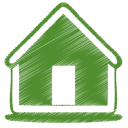BackHome
KK音標子音有二十四個。播放上一個到下一個單字的聲音時，不用按停止，程式會自動停止上一個單字的播放。

01. [ p ] = pet [pɛt] 寵物

02. [ b ] = bad [bæd] 壞的

03. [ t ] = ten [tɛn] 十

04. [ d ] = desk [dɛsk] 書桌

05. [ k ] = key [ki] 鑰匙

06. [ g ] = good [gʊd] 好的

07. [ f ] = food [fud] 食物

08. [ v ] = vest [vɛst] 花瓶

09. [ θ ] = thank [θæŋk] 謝謝

10. [ ð ] = this [ðɪs] 這；這個

11. [ s ] = seat [sit] 坐位

12. [ z ] = zoo [zu] 動物園

13. [ ʃ ] = short [ʃɔrt] 矮的；短的

14. [ ʒ ] = garage [gəˋrɑʒ] 車庫

15. [ tʃ ] = chair [tʃɛr] 椅子

16. [ dʒ ] = joke [dʒok] 笑話

17. [ l ] = leg [lɛg] 腳

18. [ r ] = red [rɛd] 紅色

19. [ m ] = mom [mɑm] 媽媽

20. [ n ] = noon [nun] 正午

21. [ ŋ ] = sing [sɪŋ] 唱歌

22. [ j ] = yes [jɛs] 是的

23. [ w ] = we [wi] 我們

24. [ h ] = hat [hæt] 帽子